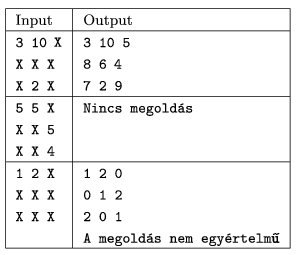Mathematical and Physical Journal
for High Schools
Issued by the MATFUND Foundation
 Already signed up? New to KöMaL?

#Problem S. 10. (September 2005)

S. 10. Write a program that fills out a 3×3 magic square if some of its entries are given. (A magic square is a square array of numbers such that the sum of numbers in any horizontal, vertical and main diagonal line is always the same number.)

The program should read the initial state of the square from the standard input. Entries of the square will be given in three lines and separated by spaces. The numbers given in advance will be integers having at most 3 digits, while unspecified entries will be denoted by an X. Your program should display the resulting magic square in the same format on the standard output. Fractions in the output should be rounded to two decimal places.

It is of course possible that the given numbers are inconsistent and the magic square can not be completed. In this case your program should report No solution''. Similarly, it may happen that there are more than one (even infinitely many) solutions. In such cases the program should choose and display one possible solution and issue a warning The solution is not unique'' in the next line.(10 pont)

Deadline expired on October 17, 2005.

Solution. It is known that all 3×3 magic squares can written as:

 x-y x+y+z x-z x+y-z x x-y+z x+z x-y-z x+y

Our task is to solve a system of linear equations. Each cell of the input which contains a number generate an equation, so the number of equations can be different from 3.

### Statistics:

 25 students sent a solution. 10 points: Engedy Balázs, Grósz Dániel, Nikházy László. 8 points: 1 student. 7 points: 1 student. 6 points: 5 students. 5 points: 3 students. 4 points: 3 students. 3 points: 1 student. 2 points: 2 students. 1 point: 1 student. 0 point: 5 students.

Problems in Information Technology of KöMaL, September 2005